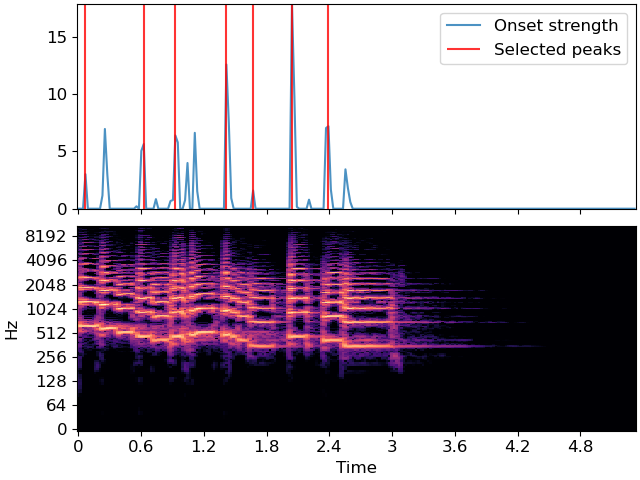# librosa.util.peak_pick¶

librosa.util.peak_pick(x, pre_max, post_max, pre_avg, post_avg, delta, wait)[source]

Uses a flexible heuristic to pick peaks in a signal.

A sample n is selected as an peak if the corresponding `x[n]` fulfills the following three conditions:

1. `x[n] == max(x[n - pre_max:n + post_max])`

2. `x[n] >= mean(x[n - pre_avg:n + post_avg]) + delta`

3. `n - previous_n > wait`

where `previous_n` is the last sample picked as a peak (greedily).

This implementation is based on 1 and 2.

1

Boeck, Sebastian, Florian Krebs, and Markus Schedl. “Evaluating the Online Capabilities of Onset Detection Methods.” ISMIR. 2012.

2

https://github.com/CPJKU/onset_detection/blob/master/onset_program.py

Parameters
xnp.ndarray [shape=(n,)]

input signal to peak picks from

pre_maxint >= 0 [scalar]

number of samples before `n` over which max is computed

post_maxint >= 1 [scalar]

number of samples after `n` over which max is computed

pre_avgint >= 0 [scalar]

number of samples before `n` over which mean is computed

post_avgint >= 1 [scalar]

number of samples after `n` over which mean is computed

deltafloat >= 0 [scalar]

threshold offset for mean

waitint >= 0 [scalar]

number of samples to wait after picking a peak

Returns
peaksnp.ndarray [shape=(n_peaks,), dtype=int]

indices of peaks in `x`

Raises
ParameterError

If any input lies outside its defined range

Examples

```>>> y, sr = librosa.load(librosa.ex('trumpet'))
>>> onset_env = librosa.onset.onset_strength(y=y, sr=sr,
...                                          hop_length=512,
...                                          aggregate=np.median)
>>> peaks = librosa.util.peak_pick(onset_env, 3, 3, 3, 5, 0.5, 10)
>>> peaks
array([  3,  27,  40,  61,  72,  88, 103])
```
```>>> import matplotlib.pyplot as plt
>>> times = librosa.times_like(onset_env, sr=sr, hop_length=512)
>>> fig, ax = plt.subplots(nrows=2, sharex=True)
>>> D = np.abs(librosa.stft(y))
>>> librosa.display.specshow(librosa.amplitude_to_db(D, ref=np.max),
...                          y_axis='log', x_axis='time', ax=ax)
>>> ax.plot(times, onset_env, alpha=0.8, label='Onset strength')
>>> ax.vlines(times[peaks], 0,
...              onset_env.max(), color='r', alpha=0.8,
...              label='Selected peaks')
>>> ax.legend(frameon=True, framealpha=0.8)
>>> ax.label_outer()
```